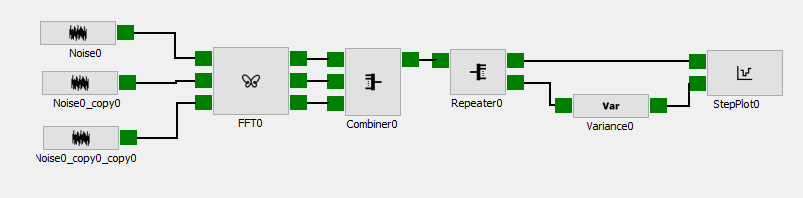Version: current

Variance

Description#

The statistics block variance calculates the variance of a dataset on either axis 0 or 1.

Input#

The input is MxN.

Output#

The output is a variance of the data on either axis 0 or 1. if the variance is calculated across axis 0 the output is 1xL, or if the variance is calculated across axis 1 the output is Kx1.

Attributes#

AttributeValue
NameVariance
Axis0
Channels1

Name#

Name specifies the name of the block.

Axis#

Axis specifies the axis of the dataset where the variance should be calculated.

Channels#

Channels specifies the number of input(s)/output(s) the block will have.

Examples#

This example 3 pink noise signal with different amplitudes, and the variance the three noise signals at each frequency point.